## Swim team members can race or dive. At a meet, 18 members race. The ratio of racers to divers is 6 : 2. How many members are on the team? Sh

Question

Swim team members can race or dive. At a meet, 18 members race. The ratio of racers to divers is 6 : 2. How many members are on the team? Show your work.

in progress 0
5 months 2021-08-14T05:01:06+00:00 1 Answers 55 views 0

## Answers ( )

24

Step-by-step explanation:

Given that:

Total number of members who race = 18

Ratio of racers to divers = 6 : 2

To find:

Total number of members of the team = ?

Solution:

Given the ratio = 6 : 2

As per the ratio given, we can let the number of racers =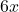and

As per the ratio given, we can let the number of divers =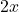As the swim team members can either race or dive, we can say that:

Total number of members on the team = Number of racers + Number of divers

Total number of members on the team =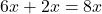As per the question statement, we are given that: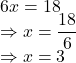Therefore, total number of team members = 8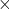3 = 24